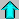# Astronomy 12 - Spring 1999 (S.T. Myers)

## Midterm 1 Review

Last update: Friday, February 5, 1999

Test Format:

You will be provided with an equation sheet, and you may also bring several sheets of your own notes. I am not asking you to memorize a bunch of equations and things, so the tests (including the final) will be open-note. Because the text does things in a different, and often confusing, way, you may not use your textbook during the exam (its not open-book).

The bulk of the exam (>75%) will be quantitative problems like in the homework and extra credit problem set. The remaining fraction of the exam will be qualitative. In Astro12, we will be talking about such a wide range of phenomena and measurements related to them that it will be critical to make sure we all know what we are talking about. Therefore, as part of each midterm (and the final) there will be some short answer questions where you will be asked to give a couple sentences explaining some topic (see the examples below).

The problems and questions below are for teaching and review purposes only. Do not hand in the answers for these, as you will not receive any credit!

Remember to bring a calculator.

I hope that these help to focus your studies...

Review Problems from the Textbook :

Some of the questions in the book can be used for review. The answers are not given, but you should have a pretty good idea if you know how to do a problem or not! You should at least have an idea on how to tackle problems like these.

1. Chapter 8: (p.177-178) Problems 3, 5, 6, 11, 12, 15, 19.

2. Chapter 11: (p.233-234) Problems 1-18, 22 (especially 2, 4, 5, 6, 11, 13, 18).

3. Chapter 12: (p.249-250) Problems 3-16 (especially 3, 6, 7, 8, 9, 10, 14, 16).

4. Chapter 13: (p.268-269) Problems 2, 13, 15, 16.

This is a list of short topical questions you should be able to answer. Some of these will be on the exam. I am looking for a sentence or two, and these are qualitative, not quantitative (read as: don't memorize numbers, just know the cartoon pictures). The key topics are in italics. It is also a good idea to look through the questions posed in the Investigations section of the on-line Lecture Notes for each lecture.

1. What is an electromagnetic wave? What are the parts of the electromagnetic spectrum and what are characteristic wavelenths for these bands?

2. What is parallax and what do we use it for?

3. What is the small-angle approximation and how do we use it?

4. What are luminosity distances and angular-diameter distances, and what are the possible differences in the values of these for the same object (if any)?

5. What is an astronomical unit? A parsec? An arcsecond? How are AU, pc and " related?

6. What is the difference between flux, flux density, luminosity, and intensity?

7. What is the UBV magnitude system?

8. What is a color quantitatively?

9. How do the observed flux and intensity of a uniform source depend on its distance from us?

10. What is a blackbody, and what is the significance of the Planck blackbody spectrum?

11. What is the Maxwell-Boltzmann distribution of gas molecules tell us?

12. How do we measure masses of stars?

13. What are visual binaries, spectroscopic binaries, and eclipsing binaries?

14. What are Kepler's Laws of Orbital Motion? Newton's Laws of Motion? Newton's Universal Gravitational Force?

15. Which of Kepler's Laws is the most important to us (or useful for us) and why?

16. What is the barycenter of a two-body system?

17. What is a Doppler shift, what is it used for, and how is it measured?

18. What is the mass function for a binary star system, and how is it different than the total or individual masses of the stars?

19. What is the significance of the Hertzsprung-Russell diagram? What quantities are plotted on the axes?

20. What is the main sequence?

21. What are the differences between spectral classes and luminosity classes of stars?

22. How can we observationally determine (or estimate) the radii of distant stars?

23. How does the main sequence lifetime of a star depend upon its mass, and why?

24. What are absorption lines and emission lines?

25. What is the Bohr model of the atom?

26. What are energy levels in the hydrogen atom?

27. What are line series and what are they called for hydrogen?

28. What is the ionization state for an atom?

29. What do the quantum numbers n,l,m,s for an electron in an atom signify?

30. What is the origin of the periodic table of the elements and how are the chemical properties of an element related to this?

31. What are molecular lines due to?Astro12 Index ---Astro12 Home

myers@dept.physics.upenn.edu Steven T. Myers